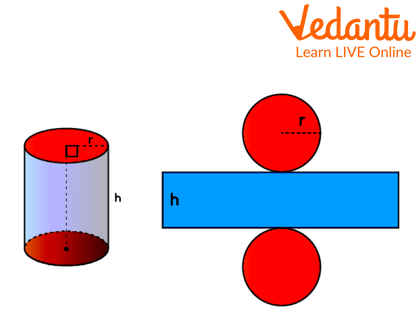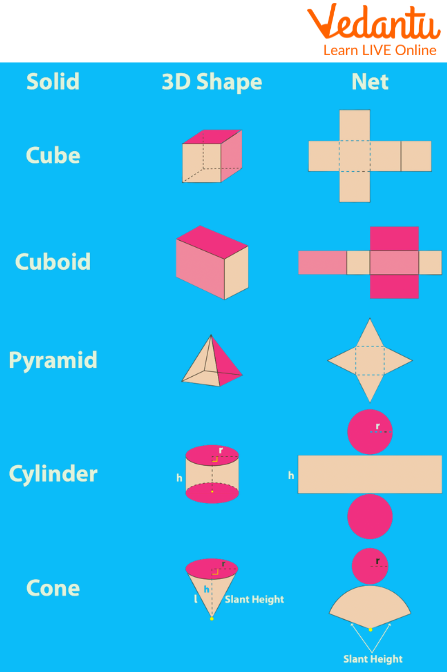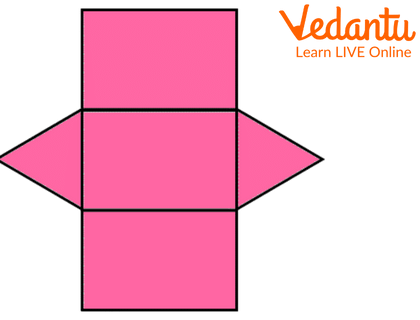Courses
Courses for Kids
Free study material
Free LIVE classes
More

# Nets of Solid ShapesLIVE
Join Vedantu’s FREE Mastercalss

## What are Solid Shapes?

Shapes can be two-dimensional or three-dimensional. A two-dimensional form (also known as a 2-D shape) has a length and a width, whereas a three-dimensional shape has a length, a breadth, and a height (also known as a 3-D shape). A solid form is a three-dimensional shape with three dimensions: length, breadth, and height (depth). Some of the solid shapes, with examples, are cuboids, spheres, cones, cubes and many more.

### What are 2D and 3D Forms of Shapes?

Two-dimensional forms are flat geometric structures composed of straight or curved lines. They have merely length and width (breadth). There is no depth in 2-D forms. 2-D forms include circles, triangles, squares, and rectangles. Polygons are shapes that are made up of three or more straight lines. Circles, ovals, triangles, squares, diamonds, rhombuses, parallelograms, and rectangles are some examples of 2-D forms.

A 3-D form, often known as a solid shape, has three dimensions: length, breadth, and height. A solid form takes up space and is supported by one or more surfaces. Solid forms include cubes, cones, spheres, and cuboids. Many real-world things have solid outlines, such as a book, laptop, ball, or birthday cone.

### What are Nets of Solid Shapes?

A solid shape's net represents its unfolded form. In other words, when we unfold and lay out the surface of a three-dimensional structure, we may see each face as a two-dimensional shape. This design is referred to as a net. The net of a cylinder, for example, is seen below. We can readily observe that it contains two 2-D forms. The first is a circle, while the second is a rectangle.Cylinder Shape Examples

### How to Make a Net For Solid Shapes?

We want to construct three-dimensional things from their nets to interpret them. A net for a three-dimensional shape is nothing more than a two-dimensional skeletal outline that, when folded, produces three-dimensional shapes.

Let's make a net of cuboids. Consider a cardboard box. Set the box flat, and cut its edges. You now have a net for that box. A net is a 2D skeleton outline, as shown in the second picture, that, when folded, results in a 3D form, as shown in the first figure.Net Of Cuboid

Given below is a table in which different shapes and their nets can be formed by cutting a sheet of paper.Solid Shapes Chart

### Conclusion

The basic difference between the solid form of an object and the 2D form of an object is that we can feel the shape of the solid object but not of the 2D object. We learned in this post that a net for a 3D shape is a 2D shape cut on a sheet of paper so that the 3D shape may be constructed. We also learnt about nets of three-dimensional solids and demonstrated how to draw a net for a cuboid.

### Sample Questions

1. A shape without any edges?

a. Sphere

b. Cube

c. Cuboid

d. Prism

Ans: Sphere

Explanation: A sphere is a solid form of the shape circle with no edges, vertices or faces.

2. This net will formNet of A Solid Image

a. Prism

b. Cylinder

c. Triangular prism

d. Cone

Ans: Triangular Prism

3. How many edges are there in a cube?

a. 8

b. 9

c. 12

d. 16

Ans: 12

Last updated date: 25th Sep 2023
Total views: 66.9k
Views today: 1.66k

## FAQs on Nets of Solid Shapes

1. Why is a crescent-shaped moon not considered a polygon?

The crescent-shaped moon is not considered a polygon as it has curved lines.

2. What shape will you get if you have the outline of a tyre and a matchbox?

If we draw the outline of a tyre, we will get a circle, and if we draw the outline of a matchbox, we will get a rectangle.

3. Which shape cannot be made out of a net?

A sphere cannot be made from the net as it does not contain a flat surface.

4. How are solid shapes visualised?

Solid forms can be represented visually in the following ways:

• By horizontally or vertically slicing or chopping them.

• By lighting a solid form.

• Consider the smallest unit from which a solid shape is formed.

5. Could a solid have several nets?

Certainly, a solid can have several nets. A cube, for example, contains 11 nets, but a cuboid has 54 nets.